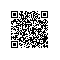# 详解 JavaScript 的私有变量

JavaScript最近有很多改进，新的语法和特性一直在添加。但有些事情不会改变，所有的东西仍然是一个对象，几乎所有的东西都可以在运行时改变，也没有公有/私有属性的概念。但是我们可以用一些技巧来改变这些，在这篇文章中，我将研究实现私有属性的各种方法

JavaScriopt 在 2015 年引入了“”这种大家都熟悉的面向对象方法，基于 C 语言的经典语言 Java 和 C# 就提供这种方法。不过很快大家就发现这些类并不是习惯的那样 —— 他们没有控制访问的属性修饰符，而且所有属性都必须定义在函数中。

class Shape {
constructor(width, height) {
this._width = width;
this._height = height;
}
get area() {
return this._width * this._height;
}
}

const square = new Shape(10, 10);
console.log(square.area);    // 100
console.log(square._width);  // 10

## WeakMap

const map = new WeakMap();

// Create an object to store private values in per instance
const internal = obj => {
if (!map.has(obj)) {
map.set(obj, {});
}
return map.get(obj);
}

class Shape {
constructor(width, height) {
internal(this).width = width;
internal(this).height = height;
}
get area() {
return internal(this).width * internal(this).height;
}
}

const square = new Shape(10, 10);
console.log(square.area);      // 100
console.log(map.get(square));  // { height: 100, width: 100 }

## Symbols

Symbol 的用法跟 WeakMap 类似。我们把 Symbol 当作实例属性的键来使用。这种方式也不会在枚举属性和 JSON.stringify 时呈现出来。这个技巧需要为每个私有属性创建 Symbol。不过，只要能访问到这些 Symbol 值，那么在类之外同样也能访问以它们为键的属性。

const widthSymbol = Symbol('width');
const heightSymbol = Symbol('height');

class Shape {
constructor(width, height) {
this[widthSymbol] = width;
this[heightSymbol] = height;
}
get area() {
return this[widthSymbol] * this[heightSymbol];
}
}

const square = new Shape(10, 10);
console.log(square.area);         // 100
console.log(square.widthSymbol);  // undefined
console.log(square[widthSymbol]); // 10

## 闭包

function Shape() {
// private vars
const this$= {}; class Shape { constructor(width, height) { this$.width = width;
this$.height = height; } get area() { return this$.width * this$.height; } } return new Shape(...arguments); } const square = new Shape(10, 10); console.log(square.area); // 100 console.log(square.width); // undefined 不过这个技巧有一点小问题。假设我们有两个不同的 Shape 对象，代码调了外面那层 Shape（函数），但返回的实例却是内部 Shape（类）的实例。多数情况下可能没什么问题，但是它会导致 square instanceof Shape 返回 false，这就是代码中潜藏的问题。 为了解决这个问题，有一种办法是将外部的 Shape 设置为其返回实例的原型： return Object.setPrototypeOf(new Shape(...arguments), this); 然而仅更改这一句话还不行，square.area 会变成未定义。get 关键字背后的工作原理是这一问题的根源。我们可以在构造器中手工指定 getter 来解决这个问题。 function Shape() { // private vars const this$ = {};

class Shape {
constructor(width, height) {
this$.width = width; this$.height = height;

Object.defineProperty(this, 'area', {
get: function() {
return this$.width * this$.height;
}
});
}
}

return Object.setPrototypeOf(new Shape(...arguments), this);
}

const square = new Shape(10, 10);
console.log(square.area);             // 100
console.log(square.width);            // undefined
console.log(square instanceof Shape); // true

function Shape() {
// private vars
const this$= {}; class Shape { constructor(width, height) { this$.width = width;
this$.height = height; } get area() { return this$.width * this\$.height;
}
}

const instance = new Shape(...arguments);
Object.setPrototypeOf(Object.getPrototypeOf(instance), this);
return instance;
}

const square = new Shape(10, 10);
console.log(square.area);             // 100
console.log(square.width);            // undefined
console.log(square instanceof Shape); // true

## 代理

const handler = {
get: function(target, key) {
if (key === '_') {
throw new Error('Attempt to access private property');
}
return target[key];
},
set: function(target, key, value) {
if (key === '_') {
throw new Error('Attempt to access private property');
}
target[key] = value;
}
};

class Shape {
constructor(width, height) {
this._width = width;
this._height = height;
}
get area() {
return this._width * this._height;
}
}

const handler = {
get: function(target, key) {
if (key === '_') {
throw new Error('Attempt to access private property');
}
return target[key];
},
set: function(target, key, value) {
if (key === '_') {
throw new Error('Attempt to access private property');
}
target[key] = value;
}
}

const square = new Proxy(new Shape(10, 10), handler);
console.log(square.area);             // 100
console.log(square instanceof Shape); // true
square._width = 200; // Error: Attempt to access private property

get: function(target, key) {
if (key === '_') {
throw new Error('Attempt to access private property');
} else if (key === 'toJSON') {
const obj = {};
for (const key in target) {
if (key !== '_') { // Only copy over the public properties
obj[key] = target[key];
}
}
return () => obj;
}
return target[key];
}

getOwnPropertyDescriptor(target, key) {
const desc = Object.getOwnPropertyDescriptor(target, key);
if (key === '_') {
desc.enumerable = false;
}
return desc;
}

class Shape {
constructor(width, height) {
this._width = width;
this._height = height;
}
get area() {
return this._width * this._height;
}
}

const handler = {
get: function(target, key) {
if (key === '_') {
throw new Error('Attempt to access private property');
} else if (key === 'toJSON') {
const obj = {};
for (const key in target) {
if (key !== '_') {
obj[key] = target[key];
}
}
return () => obj;
}
return target[key];
},
set: function(target, key, value) {
if (key === '_') {
throw new Error('Attempt to access private property');
}
target[key] = value;
},
getOwnPropertyDescriptor(target, key) {
const desc = Object.getOwnPropertyDescriptor(target, key);
if (key === '_') {
desc.enumerable = false;
}
return desc;
}
}

const square = new Proxy(new Shape(10, 10), handler);
console.log(square.area);             // 100
console.log(square instanceof Shape); // true
console.log(JSON.stringify(square));  // "{}"
for (const key in square) {           // No output
console.log(key);
}
square._width = 200;                  // Error: Attempt to access private property

class Shape {
private width;
private height;

constructor(width, height) {
this.width = width;
this.height = height;
}

get area() {
return this.width * this.height;
}
}
const square = new Shape(10, 10)
console.log(square.area); // 100

// Compile time error: Property 'width' is private and only accessible within class 'Shape'.
console.log(square.width); // 10

TypeScript 不会尝试在运行时智能地阻止访问私有属性。我只是把它列在这里，让人们意识到它并不能解决我们所看到的任何问题。你可以自己看看上面的 TypeScript 会生成什么样的 JavaScript。

## 未来

class Shape {
#height;
#width;

constructor(width, height) {
this.#width = width;
this.#height = height;
}

get area() {
return this.#width * this.#height;
}
}

const square = new Shape(10, 10);
console.log(square.area);             // 100
console.log(square instanceof Shape); // true
console.log(square.#width);           // Error: Private fields can only be referenced from within a class.使用钉钉扫一扫加入圈子
+ 订阅